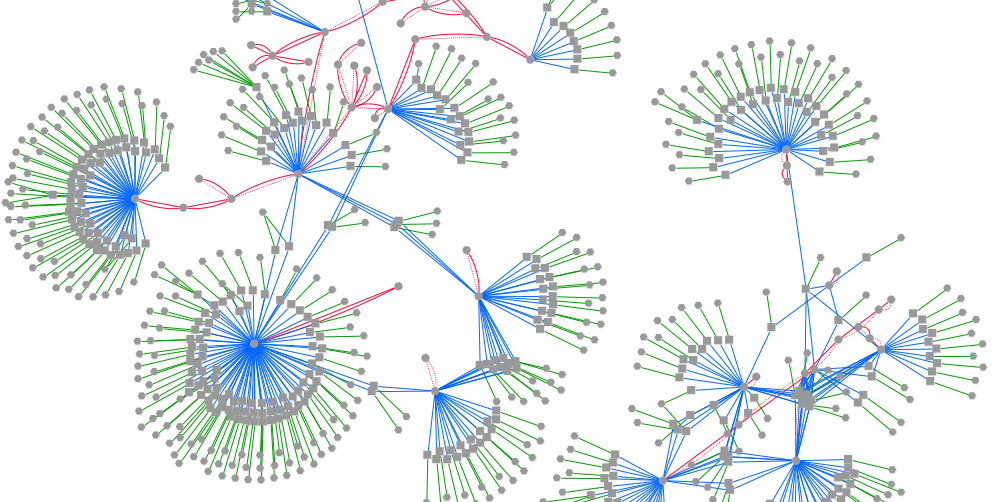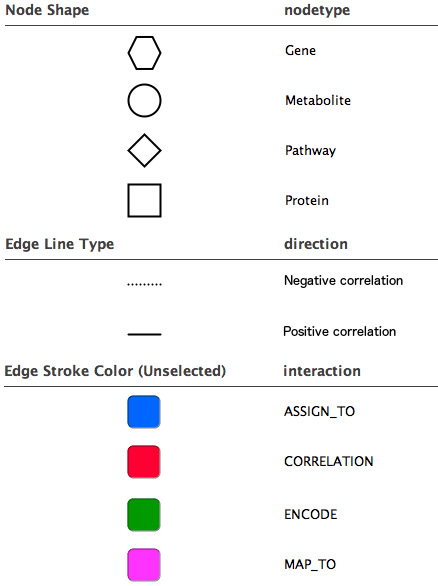## fetchDiffCorrGrinnNetwork

This example shows how to compute a differential correlation network and expand the network with information from Grinn internal database

1. INPUT

The table summarizes important arguments

ArgumentValueDescription
datX1data frame containing normalized-omic data of one conditionColumns correspond to entities e.g. genes and rows to samples. Require 'nodetype' at the first row to indicate the type of entities in each column.
datX2data frame containing normalized-omic data of another conditionIt uses the same format as datX1.
datY1data frame containing normalized-omic data of one conditionIt uses the same format as datX1. Or it can be NULL, if there is only one omic data type
datY2data frame containing normalized-omic data of another conditionIt uses the same format as datX1. Or it can be NULL, if there is only one omic data type
pDiffnumerical valueThe maximum pvalues (pvalDiff), to include edges in the output
xToentity type e.g. metaboliteThe correlation network can be expanded from datX entites to a specific entity type, by providing a value to sourceTo
yToentity type e.g. geneThe correlation network can be expanded from datY entites (if not NULL) to a specific entity type, by providing a value to yTo

2. EXECUTE FUNCTION

Compute a differential correlation network of metabolites in normal and cancer tissue and expand to the grinn network of metabolite-gene

``````
#1. load metabolomics data from a csv file
#2. show dimensions of metabolomics data
dim(datMet)
#3. show the first 10 rows and 10 columns
datMet[1:10,1:10]

#!!--- NOTE: The following codes convert kegg ids to grinn ids. If the input data is already the grinn ids, these steps can be skipped.
grinnID = convertToGrinnID(txtInput=colnames(datMet), nodetype="metabolite", dbXref="kegg") #call grinn function to convert ids
grinnID = grinnID[!duplicated(grinnID[,1]),] #keep the first mapped id
colnames(datMet) = lapply(colnames(datMet),function(x) ifelse(length(which(grinnID\$FROM_kegg == x))>0,as.character(grinnID\$GRINNID[which(grinnID\$FROM_kegg == x)]),x))
#---------- END id conversion ----------#

datMetNormal = datMet[1:40,] #normal condition
datMetTumor = rbind(nodetype=rep("metabolite"),datMet[41:79,]) #tumor condition
result <- fetchDiffCorrGrinnNetwork(datX1=datMetNormal, datX2=datMetTumor, pDiff=1e-4, method="spearman", returnAs="tab", xTo="gene")
#display the first 10 edgelists
result\$edges[1:10,]
datMet2 = datMet[c(1:7,42:50),]
datMetNormal = datMet2[1:7,]
datMetTumor = rbind(nodetype=rep("metabolite"),datMet2[8:13,])
datGeneNormal = datGene[1:7,]
datGeneTumor = rbind(nodetype=rep("gene"),datGene[8:13,])
result <- fetchDiffCorrGrinnNetwork(datX1=datMetNormal, datX2=datMetTumor, datY1=datGeneNormal, datY2=datGeneTumor, pDiff=1e-5, method="spearman", returnAs="tab", xTo="protein", yTo="pathway")

``````
3. EXPORT OUTPUT

Export the network as tab-delimited files to visualize in Cytoscape

``````
write.table(as.matrix(result\$edges),"diffcorrNwEdge.txt",sep="\t",row.names = F, quote = FALSE)
write.table(as.matrix(result\$nodes),"diffcorrNwNode.txt",sep="\t",row.names = F, quote = FALSE)
``````
4. VISUALIZATION

The figure is generated by Cytoscape 3.1.1 using grinn style (grinn.xml). It is corresponding to the cytoscape file diffcorrNw.cys.

5.Diagram legend